Next: Example 13.2: Convex mirrors Up: Paraxial Optics Previous: Worked Examples

## Example 13.1: Concave mirrors

Question: An object of height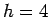cm is placed a distance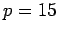cm in front of a concave mirror of focal length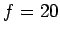cm. What is the height, location, and nature of the image? Suppose that the object is moved to a new position a distance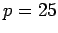cm in front of the mirror. What now is the height, location, and nature of the image?

Answer: According to Eq. (358), the image distanceis given by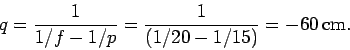Thus, the image is virtual (since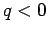), and is located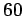cm behind the mirror. According to Eq. (352), the magnification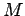of the image is given by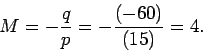Thus, the image is upright (since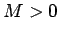), and magnified by a factor of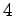. It follows that the height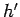of the image is given by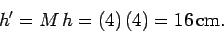If the object is moved such that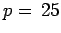cm then the new image distance is given by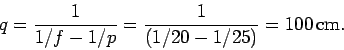Thus, the new image is real (since), and is located 100cm in front of the mirror. The new magnification is given by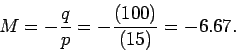Thus, the image is inverted (since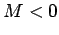), and magnified by a factor of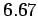. It follows that the new height of the image is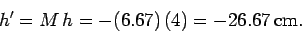Note that the height is negative because the image is inverted.Next: Example 13.2: Convex mirrors Up: Paraxial Optics Previous: Worked Examples
Richard Fitzpatrick 2007-07-14